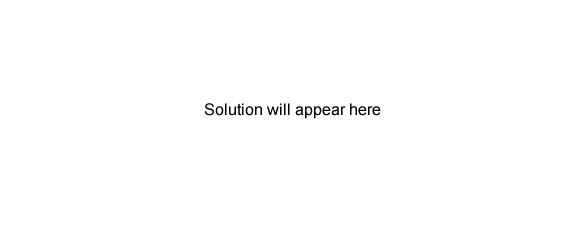Volumetric analysis exercises (2009 VCE) A student is to accurately determine the concentration of a solution of sodium hydrogencarbonate in a titration against a standard solution of hydrochloric acid, HCl. The first step in this experiment is to accurately dilute 100.0 mL of a 1.00 M HCl stock solution to a 0.100 M solution using a 1.00 L volumetric flask. However, instead of using distilled water in the dilution, the student mistakenly adds 900.0 mL of 0.0222 M sodium hydroxide, NaOH, solution. a. Write an equation for the reaction that occurs in the 1.00 L volumetric flask. Solution Calculate the concentration of the hydrochloric acid in the 1.00L volumetric flask after the student added the sodium hydroxide solution. Give your answer to correct significant figures. Solution. The student then uses this contaminated hydrochloric acid solution to determine the accurate concentration of the unknown sodium hydrogencarbonate solution. Will the calculated concentration of sodium hydrogencarbonate solution be greater or smaller than the true value? Justify your answer. Solution